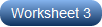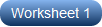# Multiplying Mixed Numbers Worksheets

Count on our printable multiplying mixed numbers worksheets for all the practice you need to perfect your skills in multiplying a mixed number with another mixed number, or multiplying three mixed numbers or completing the fraction multiplication equations. Without even realizing it, your grade 5, grade 6, and grade 7 learners will be well on their way to multiplying any number of mixed fractions with ease. It is well known that repeated exposure propels one to fluency, and these multiplying mixed numbers worksheets are sure to give you plenty of it. The process remains the same, but the difficulty level increases. Evaluation becomes easy with our answer keys. Our free multiplying mixed numbers worksheet is the first step to structured practice.

Multiplying Mixed Numbers by Mixed Numbers

Make lightning-fast progress with these multiplying mixed fractions worksheet pdfs. Change the mixed numbers to improper fractions, cross-cancel to reduce them to the lowest terms, multiply the numerators together and the denominators together and convert them to mixed numbers, if improper fractions.Finding the Product of Three Mixed Numbers

Revisiting concepts often takes the hassle out and helps 5th grade, 6th grade, and 7th grade learners spring into action in finding the product of three mixed numbers. The addition of a third term doesn't deter them.Completing the Fraction Multiplication Equation

Convert mixed fractions into improper fractions. Rearrange the equation, making the missing multiplier or multiplicand the subject, and flip the fraction when you put it on the other side of the equation and simplify it.